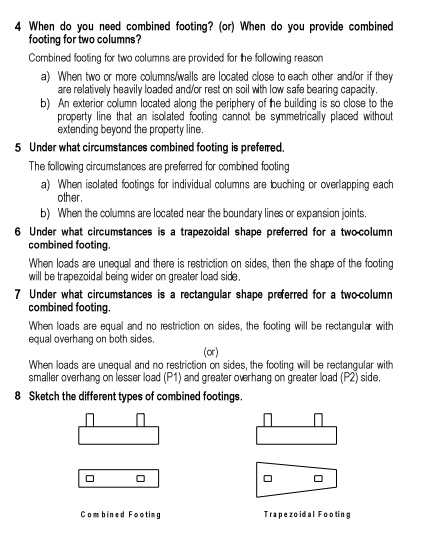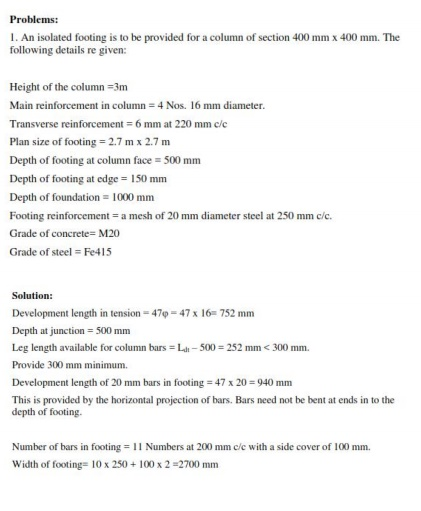Home | | Design of Reinforced Cement Concrete Elements | Design Problem, Important Question And Answer: Civil - Limit State Design Of Footing

# Design Problem, Important Question And Answer: Civil - Limit State Design Of Footing

Civil - Design of Reinforced Concrete Elements - Limit State Design Of Footing2. Design an isolated footing for an R.C. column of size 230 mm x 230 mm which carries a vertical load of 500 kN. The safe bearing capacity of soil is 200 kN/m2. Use M20 concrete and Fe 415 steel.

Solution

Step 1: Size of footing

Load on column = 600 kN

Extra load at 10% of load due to self weight of soil = 60 kN

Hence, total load, P = 660 kN

Required area of footing, - = _ /01. = _ 223433 = 5. 5_64

Assuming a square footing, the side of footing is 7 = 0 = _ )5. 5 = 8. 94_6Hence, provide a footing of size 1.85 m x 1.85 m

Net upward pressure in soil, : =   ;__   _ = 175.3_BC/E, __ < 200_BC/E, Hence O.K.

+.<=_>_+.<=

Hence, factored upward pressure of soil, pu = 263 kN/m2 and, factored load, Pu = 900 kN.

Step 2: Two way shear

Assume an uniform overall thickness of footing, D = 450 mm.

Assuming 12 mm diameter bars for main steel, effective thickness of footing 'd' is d = 450 - 50 - 12 - 6 = 382 mm

The critical section for the two way shear or punching shear occurs at a distance of d/2 from the face of the column (See Fig. 6), where a and b are the sides of the column.

Hence, punching area of footing = (a + d)2 = (0.23 + 0.382)2 = 0.375 m2 here a = b =side of column

Punching shear force = Factored load - (Factored upward pressure x punching area of footing)

=  900 - (263 x 0.375)

=  801.38 kN

3.        Design an isolated footing for an R.C. column of size 300 mm x 300 mm which carries a vertical load of 800 kN together with an uniaxial moment of 40 kN-m. The safe bearing capacity of soil is 250 kN/m2. Use M25 concrete and Fe 415 steel.

Solution

Step 1: Size of footing

Load on column = 800 kN

Extra load at 10% of load due to self weight of soil = 80 kN Hence, total load, P = 880 kN

Let us provide a square isolated footing, where L=B Equating the maximum pressure of the footing to SBC of soil,

+_ = ���l

i.e.,  99304 +_Z30_V5_2 = 4\3On solving the above equation, and taking the least and feasible value, B = 2 m

Hence, provide a square footing of size 2 m x 2 m

The maximum and minimum soil pressures are given by

T6PV = _ 93344 +_Z34_V5_2 = 453 X[64 < __250 6X[4 __�. �.T6LI = _ 93344 ?_Z34_V5_2 = 8e3 6X[4 _ y _''''____�. -.Hence, factored upward pressures of soil are,

pu,max  = 345 kN/m2  and  pu,min  = 255 kN/m2

Further, average pressure at the center of the footing is given by

pu,avg  = 300 kN/m2

and, factored load, Pu = 900 kN, factored uniaxial moment, Mu = 60 kN-m

Step 2: Two way shear

Assume an uniform overall thickness of footing, D = 450 mm

Assuming 16 mm diameter bars for main steel, effective thickness of footing 'd' is d = 450 - 50 - 16 - 8 = 376 mm

The critical section for the two way shear or punching shear occurs at a distance of d/2 from the face of the column (Fig. 9), where a and b are the dimensions of the column.

Hence, punching area of footing = (a + d)2 = (0.30 + 0.376)2 = 0.457 m2 where a = b = side of column

Punching shear force = Factored load - (Factored average pressure x punching area of footing)

=  1200 - (300 x 0.457)

=  1062.9 kN

Therefore, nominal shear stress in punching or punching shear stress ?V is computed as

Since the punching shear stress (1.05 N/mm2) is less than the allowable shear stress (1.25 N/mm2), the assumed thickness is sufficient to resist the punching shear force.

Hence, the assumed thickness of footing D = 450 mm is sufficient.

The effective depth for the lower layer of reinforcement, , d = 450 - 50 - 8 = 392 mm, and the effective depth for the upper layer of reinforcement, d = d = 450 - 50 - 16 - 8 = 376 mm.

Step 3: Design for flexure

The critical section for flexure occurs at the face of the column (Fig. 10).

The projection of footing beyond the column face is treated as a cantilever slab subjected to factored upward pressure of soil.

Factored maximum upward pressure of soil, pu,max = 345 kN/m2

Factored upward pressure of soil at critical section, pu = 306.75 kN/m2

Projection of footing beyond the column face, l = (2000 - 300)/2 = 850 mm

Bending moment at the critical section in the footing is ��

Mu = 119.11 kN-m/ m width of footing

Ast = 914.30 mm2 and 21,735.76 mm2

Selecting the least and feasible value, Ast = 914.30 mm2

The corresponding value of pt = 0.24 %

Hence from flexure criterion, pt = 0.24 %

Step 4: One way shear

The critical section for one way shear occurs at a distance of 'd' from the face of the column (Fig. 11).

Step 5: Check for

Step 6: Check for bearing stress

The load is assumed to disperse from the base of column to the base of footing at rate of 2100H:1V. at he bottom of footing =

Study Material, Lecturing Notes, Assignment, Reference, Wiki description explanation, brief detail
Civil : Design of Reinforced Concrete Elements : Limit State Design Of Footing : Design Problem, Important Question And Answer: Civil - Limit State Design Of Footing |

Related Topics